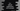# C++ isblank function explanation with example## C++ isblank() function:

isblank() function is used to check if a character is blank or not. It is defined in cctype header.

In this post, we will learn how isblank() works with examples.

### Definition of isblank:

isblank function checks for a white-space character. A white-space character can be :

• ’ ’ or space
• ‘\t’ or horizontal tab
• ‘\v’ or vertical tab
• ‘\n’ or newline
• ‘\f’ or feed
• ‘\r’ or carriage return

It is defined in header and defined as:

``int isblank(int c);``

It takes the character to check c as the parameter, casted to an integer. It returns an integer value. If it is non-zero, it is a blank character, i.e. true. Else, it returns 0.

### Example:

Let’s try to use isblank to count the number of blank spaces in a string. For example:

``````#include <iostream>
#include <cctype>

using namespace std;

int main()
{
char str;
int count = 0;
int i = 0;

cout << "Enter a string :" << endl;
cin.get(str, 100);

do
{
if (isspace(str[i]))
{
count++;
}
i++;
} while (str[i] != '\0');

cout << "Total number of blank found :  " << count << endl;
}``````

Here,

• We are calculating the total number of blanks in a user given string.
• str is used to store the user input string.
• isspace is used to check if a character is blank or not. If yes, we are incrementing the value of count.
• Finally, it is printing the value of count i.e. total blanks found.

It will print output as like below:

``````Enter a string :
hello world !!
Total number of blank found :  2``````